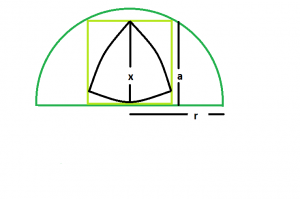# Biggest Reuleaux Triangle inscirbed within a square inscribed in a semicircle

Given here is a semicircle of radius r which inscribes a square which in turn inscribes a reuleaux triangle. The task is to find the maximum possible area of this reuleaux triangle.

Examples:

```Input : x = 5
Output : 14.0954

Input : x = 8
Output : 36.0842
```

## Recommended: Please try your approach on {IDE} first, before moving on to the solution.Approach:We know, the side of the square inscribed within a semicircle is, a = 2r/√5. (Please refer here)

Also, in the reuleaux triangle, x = a.
So, x = 2*r/√5
So, Area of Reuleaux Triangle:

```A = 0.70477*x^2 = 0.70477*(r^2/5)
```

Below is the implementation of the above approach:

## C++

 `// C++ Program to find the biggest Reuleaux triangle ` `// inscribed within in a square which in turn ` `// is inscribed within a semicircle ` ` `  `#include ` `using` `namespace` `std; ` ` `  `// Function to find the biggest reuleaux triangle ` `float` `Area(``float` `r) ` `{ ` ` `  `    ``// radius cannot be negative ` `    ``if` `(r < 0) ` `        ``return` `-1; ` ` `  `    ``// height of the reuleaux triangle ` `    ``float` `x = (2 * r) / ``sqrt``(5); ` ` `  `    ``// area of the reuleaux triangle ` `    ``float` `A = 0.70477 * ``pow``(x, 2); ` ` `  `    ``return` `A; ` `} ` ` `  `// Driver code ` `int` `main() ` `{ ` `    ``float` `r = 5; ` `    ``cout << Area(r) << endl; ` ` `  `    ``return` `0; ` `} `

## Java

 `// Java Program to find the biggest  ` `// Reuleaux triangle inscribed within ` `// in a square which in turn is  ` `// inscribed within a semicircle ` `import` `java.lang.Math; ` ` `  `class` `GFG ` `{ ` ` `  `// Function to find the biggest reuleaux triangle ` `static` `float` `Area(``float` `r) ` `{ ` ` `  `    ``// radius cannot be negative ` `    ``if` `(r < ``0``) ` `        ``return` `-``1``; ` ` `  `    ``// height of the reuleaux triangle ` `    ``float` `x = (``2` `* r) /(``float``)(Math.sqrt(``5``)); ` ` `  `    ``// area of the reuleaux triangle ` `    ``float` `A = ``0``.70477f *(``float``)(Math.pow(x, ``2``)); ` ` `  `    ``return` `A; ` `} ` ` `  `// Driver code ` `public` `static` `void` `main(String[] args) ` `{ ` `    ``float` `r = ``5``; ` `    ``System.out.println(Area(r)); ` `} ` `} ` ` `  `// This code is contributed by Mukul Singh. `

## Python3

 `# Python3 Program to find the biggest  ` `# Reuleaux triangle inscribed within  ` `# in a square which in turn is inscribed  ` `# within a semicircle ` `import` `math as mt ` ` `  `# Function to find the biggest ` `# reuleaux triangle ` `def` `Area(r): ` ` `  `    ``# radius cannot be negative ` `    ``if` `(r < ``0``): ` `        ``return` `-``1` ` `  `    ``# height of the reuleaux triangle ` `    ``x ``=` `(``2` `*` `r) ``/` `mt.sqrt(``5``) ` ` `  `    ``# area of the reuleaux triangle ` `    ``A ``=` `0.70477` `*` `pow``(x, ``2``) ` ` `  `    ``return` `A ` ` `  `# Driver code ` `r ``=` `5` `print``(Area(r)) ` ` `  `# This code is contributed by ` `# Mohit Kumar 29 `

## C#

 `// C# Program to find the biggest  ` `// Reuleaux triangle inscribed within ` `// in a square which in turn is  ` `// inscribed within a semicircle ` `using` `System; ` ` `  `class` `GFG ` `{ ` ` `  `// Function to find the biggest reuleaux triangle ` `static` `double` `Area(``double` `r) ` `{ ` ` `  `    ``// radius cannot be negative ` `    ``if` `(r < 0) ` `        ``return` `-1; ` ` `  `    ``// height of the reuleaux triangle ` `    ``double` `x = (2 * r) / (``double``)(Math.Sqrt(5)); ` ` `  `    ``// area of the reuleaux triangle ` `    ``double` `A = 0.70477  * (``double``)(Math.Pow(x, 2)); ` ` `  `    ``return` `A; ` `} ` ` `  `// Driver code ` `public` `static` `void` `Main() ` `{ ` `    ``double` `r = 5; ` `    ``Console.WriteLine(Area(r)); ` `} ` `} ` ` `  `// This code is contributed by chandan_jnu `

## PHP

 ` ` ` `

Output:

```14.0954
```

Attention reader! Don’t stop learning now. Get hold of all the important DSA concepts with the DSA Self Paced Course at a student-friendly price and become industry ready.

My Personal Notes arrow_drop_upBudding Web DeveloperKeen learnerAverage CoderDancer&Social Activist

If you like GeeksforGeeks and would like to contribute, you can also write an article using contribute.geeksforgeeks.org or mail your article to contribute@geeksforgeeks.org. See your article appearing on the GeeksforGeeks main page and help other Geeks.

Please Improve this article if you find anything incorrect by clicking on the "Improve Article" button below.